If you use formatted variables in a CLASS statement in procedures such as MEANS or UNIVARIATE, SAS will use the formatted values of those variables and not the internal values. For example, consider you have a data set (Health) with variables Subj, Age, Weight, and Height. You want to see the mean Weight and Height for three age groups: 0 to 40, 41-70, and greater than 70. A program to create the Health data set and compute the means is shown below.

```Data Health; input Subj \$ Age Weight Height; datalines; 001 23 120 72 002 35 200 80 003 77 98 63 004 51 230 75 005 15 98 54 006 44 160 68 007 83 111 65 008 10 . 45 009 60 200 . ; proc format; value AgeGrp 0-40 = '0 to 40' 41-70 = '41 to 70' 71-high = '&gt;70'; run;   proc means data=Health n nmiss mean; class Age; format Age AgeGrp.; var Weight Height; run;```

Here is the output: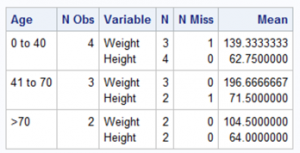Notice that there was no need to run another DATA step and create a variable representing age ranges. Not only is this an easier way to get these results—it is also much more efficient.

The other day, I received an email from a person and he posed the following question. How can I see the mean of the variable Sales (in the SASHELP data set Shoes) for three ranges of Sales: 0 to 100,000; 100,000 to 200,000; 200,000 and above?

My first thought was to create a variable (called Range) and then use that variable in a CLASS statement with PROC MEANS. However, after some thought, I wondered if I could use the Sales variable in the CLASS statement (and supply a format) and use the same variable in the VAR statement. As is often the case, the best way to answer a SAS programming question is to write a short program and see what happens. Here is what I suggested:

```proc format; value SalesRange 0 - &lt;100000 = 'Lower' 100000 - &lt;200000 = 'Middle' 200000 - high = 'Upper'; run;   proc means data=sashelp.Shoes n nmiss mean std; class Sales; format Sales SalesRange.; var Sales; run;```

Here is the output: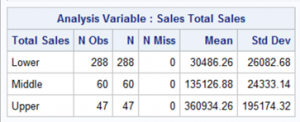As you can see from the output, it worked just fine.

Before we leave this topic, let's use the first example, referencing the Health data set, to demonstrate a Multi-Label Format. Suppose you want to see means of Weight and Height for two different ranges of Age. One range is the original 0–40, 41–70, and 70+; the other is 0–50 and 51+. Here is a way to accomplish this using a single format:

```proc format; value AgeGrp (multilabel) 0-40 = '0 to 40' 41-70 = '41 to 70' 71-high = '&gt;70'   0-50 = '0 to 50' 51-high = '&gt;50'; run;   proc means data=Health n nmiss mean; class Age / MLF; format Age AgeGrp.; var Weight Height; run;```

You need to add the option "multilabel" following the format name on the VALUE statement.  Without this option, you will get an error message if you have overlapping ranges. Next, use the option MLF (multi-label format) on the CLASS statement. Here is the output: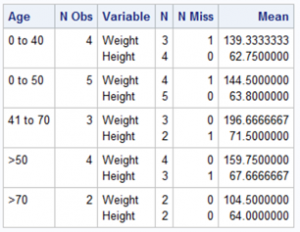You now have the mean Weight and Height for two separate ranges of Age.

Consider using a formatted variables in CLASS statements for those procedures that allow this statement. It will save you time and reduce CPU time as well.

If you liked this blog, then you might like my latest book: Cody’s Collection of SAS Blogs. It gathers all of my best tips and tricks from my blogs in one place for easy reference.

Using formatted CLASS variables was published on SAS Users.

In my previous blog, you saw how to create a Beale cipher. In this blog, you will see a program that can decode a Beale cipher. As a reminder, here is a list of numbers that you can use as a substitute for a letter when creating your cipher.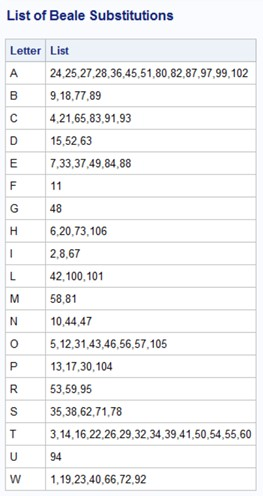Now, suppose you want to send the following message: "Come to safe house at ten tonight." One possible cipher for this message is:

```65 12 81 84 55 46 3 73 88 71 80 11 7
20 57 94 35 84 82 22 29 33 44 16 31 10
67 48 73 60```

The first step to decode this cipher is the same as the first step in the program to create the cipher: Make a list of possible numbers to represent each letter. I'll repeat it here:

```*Create the list of letters and numbers; Data Decipher; length Letter \$ 1; infile 'c:\Books\Blogs\Declare.txt'; input Letter : \$upcase1. @@; N + 1; output; run;   title "Listing of Data Set Decipher"; title2 "First Five Observations"; proc print data=Decipher(obs=5) noobs; run;```

This is the program that created the list of numbers corresponding to each letter. The next step in the program to create a Beale cipher was to sort by Letter. This time you want it in number order. Because it is already in order by the variable N, you don't have to sort it. Here are the first five observations in data set Decipher: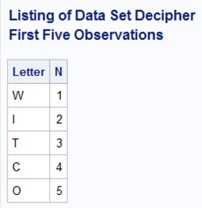The next step is to read the message and make a SAS data set.

```*Make a SAS data set from the Message text; data Message; infile 'c:\books\Blogs\Cipher\Message.txt'; input NN @@; run;   title "First 5 Observations from Data Set Message"; proc print data=Message(obs=5) noobs; run;```

The final step is to create a temporary array (long enough to hold all the numbers). Each element in this array will contain the letter corresponding to the position in the array. The DATA step below first loads the temporary array elements with the appropriate letters and then reads each number from the file Message.txt (that contains the secret code). The temporary array is acting as a lookup table to find the letter corresponding to the number. I have annotated the program so that you can see exactly what is going on.

```data Final; length Letter \$ 1 String \$ 200; array Letters \$ _temporary_; ❶ set Decipher (keep=Letter) end=Last_Obs; ❷ N+1; Letters[N] = Letter; ❸ if Last_Obs then do i = 1 to N_Message; ❹ set Message Nobs=N_Message; ❺ Letter = Letters[NN]; ❻ String = catx(' ',String,Letter); ❼ if i = N_Message then output; ❽ end; keep String; run;   title "Decoded message"; proc print data=Final noobs; ❾ run;```

❶ Create a temporary array. Each element in the temporary array (Letters) is a letter corresponding the element number. For example, Letters is 'W', Letters is 'I', and so forth.

❷ Bring in the observations in data set Decipher. Each observation in this data set contains the first letter of each word in the document. The END= option lets you know when you have read the last observation in the Decipher data set.

❸ Load up the temporary array based on the values of N and Letter

❹ Once the temporary array is loaded, read in the observations in data set Message. Notice that the variable N_Message was set to the number of observations in data set Message at compile time by using the SET option NOBS=.

❺ Bring in the observations from data set Message.

❻ Decipher the number (NN) to determine the letter it represents.

❼ Use the CATX function to add all the letters to the variable String.

❽ After all the numbers from the file Message.txt have been processed, it is time to output an observation containing the variable String.

❾ Use PROC PRINT to print out the message.

I showed this program to my friend Mark Jordan (aka SAS Jedi), and he came up with a solution that uses formats to do the table lookup. It is probably an easier and more elegant program than mine (his programs usually are), and I am including his program here.

The first step is once again to create the cipher. Make a list of possible numbers to represent each letter.  This time, though, we’ll create the Decipher data set so that it can be used to build a SAS format.

```*Create the list of letter and numbers; data Decipher; retain Fmtname 'Decipher' Type 'N'; ❶ length LABEL \$ 1; ❷ infile 'c:\Books\Blogs\Declare.txt'; input Label : \$upcase1. @@; N + 1; Start=N; ❸ output; ❹ drop N; run;   title "Listing of Data Set Decipher"; title2 "First 5 Observations"; proc print data=Decipher(obs=5) noobs; ❺ run;```

❶ FMTNAME and TYPE are required to be the same value for each observation. We accomplish that with a RETAIN statement.

❷ LABEL and START are the other two required variables for a PROC FORMAT control data set.

❸ Set Start to N.

❹ Write one row for each value we want to decode.

❺ Print the first 5 observations of the Decipher data set.

The next step is to create a format from the Decipher data set:

```* Make a format from the Decipher data set; proc format cntlin=Decipher fmtlib; run;```

The FMTLIB option produces a report documenting the format. Here is a sample: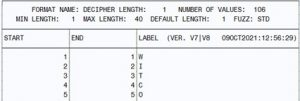The final step is to use the format on each number in the message text to decode it. The first DATA step below reads each number from the file Message.txt (that contains the secret code) to create the Message data set. The second DATA step reads the Message data set and applies the format to each numeric value using the PUT function. This produces the letter corresponding to the number. I have annotated the program so that you can see exactly what is going on.

```*Make a SAS data set from the Message text; data Message; infile 'c:\Books\Blogs\Cipher\Message.txt'; input NN @@; run;   data Final; length String \$200; retain string; keep String; set Message end=last; ❶ String = catx(' ',String,put(NN,decipher.)); ❷ if last then output; run;   title "Decoded message"; proc print data=Final noobs; ❸ run;```

❶ Bring in the observations from the Message data set.
❷ Use the PUT function to produce the correct letter, and the CATX function to combine the letters into the variable String.
❸ Use PROC PRINT to print out the message.

I hope you enjoy both of these programs. Please add a comment to the blog with your preference. I think I'll vote for Mark's program!

Fun with Ciphers (Part 2) was published on SAS Users.

This blog serves two purposes: the main purpose is to show you some useful SAS coding techniques, and the second is to show you an interesting method of creating a Beale cipher.

TJ Beale is famous in Virginia for leaving behind three ciphers, supposedly describing the location of hidden gold and treasures. (Most cryptologists and historians believe the whole set of ciphers and treasure was a hoax.) In one of the ciphers, he used a method based on the Declaration of Independence. His coding method was as follows:

• Get a copy of the Declaration of Independence and number each word.
• Take the first letter of each word and form a list.
• Associate each number with that letter.

For example, consider this text:

“Four score and seven years ago, our fathers brought forth upon this continent a new nation, conceived in liberty and dedicated to the proposition that all men are created equal. “

To create a Beale cipher, you would proceed as follows:

Four(1) score(2) and(3) seven(4) years(5) ago(6), our(7) fathers(8) brought(9) forth(10) upon(11) this(12) continent(13) a(14) new(15) nation(16), conceived(17) in(18) liberty(19) and(20) dedicated(21) to(22) the(23) proposition(24) that(25) all(26) men(27) are(28) created(29) equal(30).

Next, you would make a table like this:

 Letter Numbers F 1,8,10 (all the numbers of words that begin with 'F') S 2,4 A 3,6,14,20,26,28 Y 5 …and so on

You would then want to put the list in alphabetical order like this:

 A 3,6,14,20,26,28 B 9 C 13,17,29 D 21 E 30 F 1,8,10 …and so on

To create your cipher, select any number at random from the list of numbers, corresponding to the letter that you want to encode. The advantage of this over a simple substitution cipher is that you cannot use frequency analysis to guess what letter a particular number represents.

This blog explains how to create a Beale cipher; my next blog will explain how to decipher a Beale cipher.

You need to start out with a book or document that is accessible to the sender and recipient of the cipher. To offer some additional security, you could decide to start from a specific page in a book. For a simple demonstration of how to create a Beale cipher, I have entered part of the Declaration of Independence in a text file called Declare.txt.

A funny aside: I was teaching my Functions course in the UK, in a small town north of London on the Thames. One of the programs demonstrating several SAS character functions was the program I'm using here to demonstrate how to create a Beale cipher. I had completely forgotten that the document was the Declaration of Independence. Whoops! I asked the class, "I hope you're not still angry with us about that." Apparently not, and we all had a good laugh.

Back to the problem. I will break down the program into small steps and provide a partial listing of data sets along the way, so that you can see exactly how the program works. The first step is read the text file, extract the first letter from each word, change the letter to uppercase, and associate each letter with the count of words in the text.

Here is the first part of the program.

```data Beale; length Letter \$ 1; infile 'c:\Books\Blogs\Declare.txt'; input Letter : \$upcase1. @@; ❶ N + 1; ❷ output; run;   title "First Step in the Beale Cipher (first 10 observations)"; proc print data=Beale(obs=10) noobs; run;```

❶ By using the \$UPCASE1. informat, you are selecting the first letter of each word and converting it to uppercase. If you are unfamiliar with the \$UPCASEn. informat, it is similar to the \$n. informat with the additional task of converting the character(s) to uppercase.

❷ You use a SUM statement to associate each letter with the word count.

Here is the listing from this first step: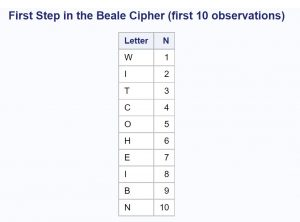Next, you need to sort the data set by Letter so that all the words that start with As, Bs, and so forth are placed together.

```proc sort data=Beale; by Letter; run;   title "The list in sorted order (partial listing)"; proc print data=Beale(obs=10) noobs; run;```

Below is a partial listing of the sorted file: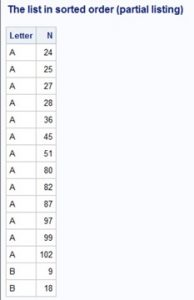Any of the numbers 24, 25, 27, and so forth can be used to code an 'A'.

The final step is to list all the letters from A to Z (Z is pronounced Zed in the UK and Canada) in a line, followed by all the possible numbers associated with each letter.

```data Next; length List \$ 40; ❸ retain List; ❹ set Beale; by Letter; ❺ if first.Letter then List = ' '; ❻ List = catx(',',List,N); ❼ if last.Letter then output; ❽ run;   title "List of Beale Substitutions"; proc print data=next(obs=5) noobs; var Letter List; run;```

❸ The variable List will hold all the possible numbers that can be used to code any of the letters. In a real program, this list might be longer.

❹ You need to RETAIN this variable; otherwise, it would be set back to a missing value for each iteration of the DATA step.

❺ Following the SET statement with a BY statement creates the two temporary variables, First.Letter and Last.Letter. First.Letter is true when you are reading the first observation for each letter—Last.Letter is true when you are reading the last observation for a letter.

❻ For the first A, B, C, and so on, initialize the variable List to a missing value.

❼ Use the CATX function to concatenate all the numbers, separated by commas.

❽ When you are done reading the last A, B, C, and so on, output the string.

Below are a few lines generated by this program: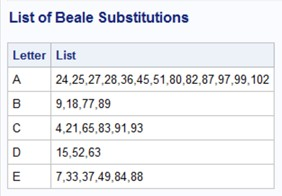For more information about the CATX function and other SAS functions, please take a look at my book, SAS Functions by Example, Second Edition.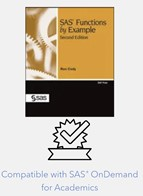Fun with Ciphers (Part 1) was published on SAS Users.

Many SAS programmers use macros. I have seen students in my SAS classes use several methods to activate their macros. One way is to load the macro in the Display manager or editor in SAS OnDemand for Academics and submit it. Another technique is to use the statement %Include macro-name.

The best way to make all of your macros available in every SAS session is to take advantage of the Auto Call facility. There are several steps to make this work. I will show you how this works in SAS running on your local computer or SAS OnDemand for Academics.

### Instructions for SAS on your local computer

First, place the macros that you plan to use in a pre-defined place, such as "C:\MySASFiles\MyMacros."

Next, edit your Autoexec.sas file. Remember, all the statements in this file are executed every time you open a SAS session. Add the following statements:

`filename MyMacros 'C:\MySASFiles\MyMacros;'`

(or wherever you placed your macros)

`options mautosource SASAutos=(MyMacros);`

(The name in parentheses is the file name above.)

You are done. The next time you open a SAS session on your local machine any of the macros in your macro library are available to use.

### Instructions for SAS OnDemand for Academics

Open SAS Studio. In the Navigation Pane, click Server Files and Folders. Create a new folder as shown below. (The new folder icon is circled.)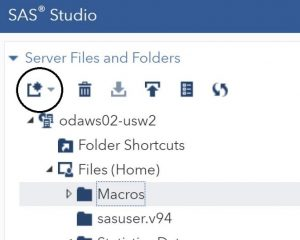In this example, I chose Macros as my folder name.

Click the Upload icon (circled in the figure below).Upload the macros from your local computer to this folder (shown below).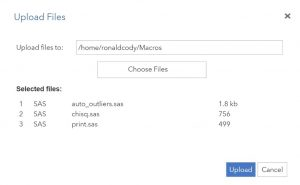Now it's time to add the appropriate statements to the Autoexec.sas file. Do this by clicking the icon circled in the figure below.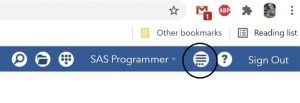Choose Edit Autoexec File from the drop-down list.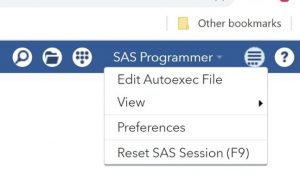Add the two lines in the box shown in the next figure.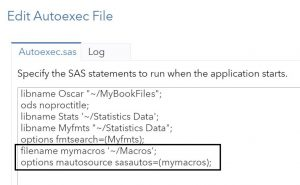Click SAVE. (You can click RUN first and check the log to see that there are any errors.)

### Demonstrating a Macro Call (with the Auto Call facility)

You can now use any of the macros in your macro folder. For example, I have a macro called %Print that will either print an entire data set or the first “n” observations and add a title to the listing. The program below was run after opening a new session in SAS OnDemand for Academics.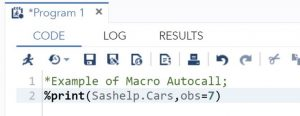Here is the result: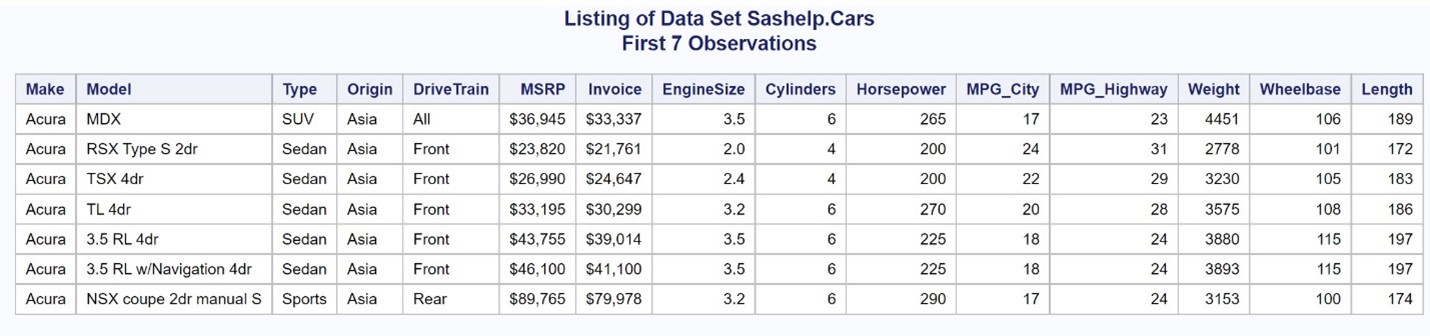If you have never used the Auto Call facility, you now know how to do it. One important final point: you must save your macros using the same name as the macro name. For example, if you have a macro called Print, save it as Print.sas. By doing that, SAS knows where to find and compile the macro that you want to use.

If you are curious about my %Print macro or want to test out the Auto Call facility, here is a listing:

```*----------------------------------------------------------------* | Program Name: Print.sas in C:\sasdata\macros | | Purpose: Macro that prints out a data set and uses the data | | set name in the title | | Calling Argument: %PRINT(dsn,obs=) | | Example: %PRINT(test,obs=5) | | Date: January 13, 1998 | | Updated March 6, 2000 to add obs positional parameter | *----------------------------------------------------------------*; %macro Print(dsn,obs=max); proc print data=&amp;dsn (obs=&amp;obs) noobs; title "Listing of data set %upcase(&amp;dsn)"; %if &amp;obs ne max %then title2 "First &amp;obs Observations";; run; %mend print;```

How to use the SAS Auto Call facility was published on SAS Users.

Last year, I wrote a blog demonstrating how to use the %Auto_Outliers macro to automatically identify possible data errors. This blog demonstrates a different approach—one that is useful for variables for which you can identify reasonable ranges of values for each variable. For example, you would not expect resting heart rates below 40 or over 100 or adult heights below 45 inches or above 84 inches. Although values outside those ranges might be valid values, it would be a good idea to check those out-of-range values to see if they are real or data errors.

In the third edition of my book, Cody's Data Cleaning Techniques, I present two macros: %Errors and %Report. These two macros provide a consolidated error report.

To demonstrate these two macros, I created a data set called Patients. A listing of the first 10 observations is shown below: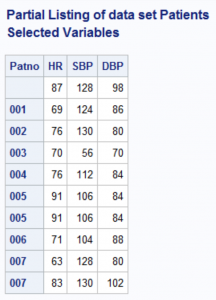Notice that the unique identifier is called Patno, and you see three variables HR (heart rate), SBP (systolic blood pressure), and DBP (diastolic blood pressure).

The calling arguments for the %Errors macro are:

• VAR=     A variable for which you have pre-defined bounds
• Low=     The lowest reasonable value for this variable
• High=    The highest reasonable value for this variable
• Missing=Error or Missing=Ignore. The default for this argument is IGNORE, but it is still good practice to include it in the call so that anyone reading your program understands how missing values are being handled.

Because you might be calling this macro for many variables, the values of two macro variables &Dsn (data set name) and &IDVar (the identifying variable such as Patno or Subj) are assigned values once, using two %Let statements. You can then call the %Errors macro for each variable of interest. When you are finished, call the %Report macro to see a consolidated report of your possible errors.

Here is an example:

```/* Set values for &amp;Dsn and %IDVar with %LET statements */ %let Dsn = Clean.Patients; %let Idvar = Patno;   %Errors(Var=HR, Low=40, High=100, Missing=error) %Errors(Var=SBP, Low=80, High=200, Missing=ignore) %Errors(Var=DBP, Low=60, High=120)   /* When you are finished selecting variables, create the report */ %Report```

You are reporting all heart rates below 40 or above 100 (and considering missing values as errors); values of SBP below 80 or above 200 (and ignoring missing values); and values of DBP below 60 or above 120 (also ignoring missing values—using the default value of IGNORE).

Here is the result:Notice that several patients with missing values for HR are flagged as errors. I should point out that I have violated a cardinal rule of macro programming: never write a macro that has no arguments—it should be a program. However, I liked the idea of calling %Errors and then %Report. Shown below are the two macros:

```/**************************************************************** | PROGRAM NAME: ERRORS.SAS in c:\Books\Cleans\Patients | | PURPOSE: Accumulates errors for numeric variables in a SAS | | data set for later reporting. | | This macro can be called several times with a | | different variable each time. The resulting errors | | are accumulated in a temporary SAS data set called | | Errors. | | ARGUMENTS: Dsn= - SAS data set name (assigned with a %LET) | | Idvar= - Id variable (assigned with a %LET) | | | | Var = The variable name to test | | Low = Lowest valid value | | High = Highest valid value | | Missing = IGNORE (default) Ignore missing values | | ERROR Missing values flagged as errors | | | | EXAMPLE: %let Dsn = Clean.Patients; | | %let Idvar = Patno; | | | | %Errors(Var=HR, Low=40, High=100, Missing=error) | | %Errors(Var=SBP, Low=80, High=200, Missing=ignore) | | %Errors(Var=DBP, Low=60, High=120) | | Test the numeric variables HR, SBP, and DBP in data | | set Clean.patients for data outside the ranges | | 40 to 100, 80 to 200, and 60 to 120 respectively. | | The ID variable is PATNO and missing values are to | | be flagged as invalid for HR but not for SBP or DBP. | ****************************************************************/ %macro Errors(Var=, /* Variable to test */ Low=, /* Low value */ High=, /* High value */ Missing=IGNORE /* How to treat missing values */ /* Ignore is the default. To flag */ /* missing values as errors set */ /* Missing=error */); data Tmp; set &amp;Dsn(keep=&amp;Idvar &amp;Var); length Reason \$ 10 Variable \$ 32; Variable = "&amp;Var"; Value = &amp;Var; if &amp;Var lt &amp;Low and not missing(&amp;Var) then do; Reason='Low'; output; end;   %if %upcase(&amp;Missing) ne IGNORE %then %do; else if missing(&amp;Var) then do; Reason='Missing'; output; end; %end;   else if &amp;Var gt &amp;High then do; Reason='High'; output; end; drop &amp;Var; run;   proc append base=Errors data=Tmp; run;   %mend Errors;```

The basic idea for the %Errors macro is to test each variable and, if it is a possible error, use PROC APPEND to add it to a data set called Errors. When the first error is detected, PROC APPEND creates the data set Errors. From then on, each observation in data set Tmp is added to data set Errors.

Most of this macro is straightforward. For those readers who are not that comfortable with macro programming, the third section (beginning with %if %upcase(&Missing)) is executed only when the value of the macro variable &Missing is not equal to IGNORE.

Below is a listing of the %Report macro:

```%macro Report; proc sort data=Errors; by &amp;Idvar; run;   proc print data=Errors; title "Error Report for Data Set &amp;Dsn"; id &amp;Idvar; var Variable Value Reason; run;   proc delete data=Errors Tmp; run;   %mend Report;```

The %Report macro is mainly a PROC PRINT of the temporary data set Errors. I added PROC DELETE to delete the two temporary data sets Error and Tmp.

You can cut and paste these macros, or you can download all of the macros, programs, and data sets from Cody's Data Cleaning Techniques Using SAS®, Third Edition, by going to support.sas.com/Cody, search for the book, then click Example Code and Data. You do not have to buy the book to download all the files (although I would be delighted if you did). This is true for all of my books published by SAS Press.

Comments and/or corrections are always welcome.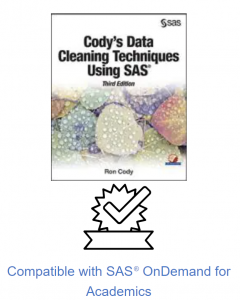Two macros for detecting data errors was published on SAS Users.

Last year, I wrote a blog demonstrating how to use the %Auto_Outliers macro to automatically identify possible data errors. This blog demonstrates a different approach—one that is useful for variables for which you can identify reasonable ranges of values for each variable. For example, you would not expect resting heart rates below 40 or over 100 or adult heights below 45 inches or above 84 inches. Although values outside those ranges might be valid values, it would be a good idea to check those out-of-range values to see if they are real or data errors.

In the third edition of my book, Cody's Data Cleaning Techniques, I present two macros: %Errors and %Report. These two macros provide a consolidated error report.

To demonstrate these two macros, I created a data set called Patients. A listing of the first 10 observations is shown below:Notice that the unique identifier is called Patno, and you see three variables HR (heart rate), SBP (systolic blood pressure), and DBP (diastolic blood pressure).

The calling arguments for the %Errors macro are:

• VAR=     A variable for which you have pre-defined bounds
• Low=     The lowest reasonable value for this variable
• High=    The highest reasonable value for this variable
• Missing=Error or Missing=Ignore. The default for this argument is IGNORE, but it is still good practice to include it in the call so that anyone reading your program understands how missing values are being handled.

Because you might be calling this macro for many variables, the values of two macro variables &Dsn (data set name) and &IDVar (the identifying variable such as Patno or Subj) are assigned values once, using two %Let statements. You can then call the %Errors macro for each variable of interest. When you are finished, call the %Report macro to see a consolidated report of your possible errors.

Here is an example:

```/* Set values for &amp;Dsn and %IDVar with %LET statements */ %let Dsn = Clean.Patients; %let Idvar = Patno;   %Errors(Var=HR, Low=40, High=100, Missing=error) %Errors(Var=SBP, Low=80, High=200, Missing=ignore) %Errors(Var=DBP, Low=60, High=120)   /* When you are finished selecting variables, create the report */ %Report```

You are reporting all heart rates below 40 or above 100 (and considering missing values as errors); values of SBP below 80 or above 200 (and ignoring missing values); and values of DBP below 60 or above 120 (also ignoring missing values—using the default value of IGNORE).

Here is the result:Notice that several patients with missing values for HR are flagged as errors. I should point out that I have violated a cardinal rule of macro programming: never write a macro that has no arguments—it should be a program. However, I liked the idea of calling %Errors and then %Report. Shown below are the two macros:

```/**************************************************************** | PROGRAM NAME: ERRORS.SAS in c:\Books\Cleans\Patients | | PURPOSE: Accumulates errors for numeric variables in a SAS | | data set for later reporting. | | This macro can be called several times with a | | different variable each time. The resulting errors | | are accumulated in a temporary SAS data set called | | Errors. | | ARGUMENTS: Dsn= - SAS data set name (assigned with a %LET) | | Idvar= - Id variable (assigned with a %LET) | | | | Var = The variable name to test | | Low = Lowest valid value | | High = Highest valid value | | Missing = IGNORE (default) Ignore missing values | | ERROR Missing values flagged as errors | | | | EXAMPLE: %let Dsn = Clean.Patients; | | %let Idvar = Patno; | | | | %Errors(Var=HR, Low=40, High=100, Missing=error) | | %Errors(Var=SBP, Low=80, High=200, Missing=ignore) | | %Errors(Var=DBP, Low=60, High=120) | | Test the numeric variables HR, SBP, and DBP in data | | set Clean.patients for data outside the ranges | | 40 to 100, 80 to 200, and 60 to 120 respectively. | | The ID variable is PATNO and missing values are to | | be flagged as invalid for HR but not for SBP or DBP. | ****************************************************************/ %macro Errors(Var=, /* Variable to test */ Low=, /* Low value */ High=, /* High value */ Missing=IGNORE /* How to treat missing values */ /* Ignore is the default. To flag */ /* missing values as errors set */ /* Missing=error */); data Tmp; set &amp;Dsn(keep=&amp;Idvar &amp;Var); length Reason \$ 10 Variable \$ 32; Variable = "&amp;Var"; Value = &amp;Var; if &amp;Var lt &amp;Low and not missing(&amp;Var) then do; Reason='Low'; output; end;   %if %upcase(&amp;Missing) ne IGNORE %then %do; else if missing(&amp;Var) then do; Reason='Missing'; output; end; %end;   else if &amp;Var gt &amp;High then do; Reason='High'; output; end; drop &amp;Var; run;   proc append base=Errors data=Tmp; run;   %mend Errors;```

The basic idea for the %Errors macro is to test each variable and, if it is a possible error, use PROC APPEND to add it to a data set called Errors. When the first error is detected, PROC APPEND creates the data set Errors. From then on, each observation in data set Tmp is added to data set Errors.

Most of this macro is straightforward. For those readers who are not that comfortable with macro programming, the third section (beginning with %if %upcase(&Missing)) is executed only when the value of the macro variable &Missing is not equal to IGNORE.

Below is a listing of the %Report macro:

```%macro Report; proc sort data=Errors; by &amp;Idvar; run;   proc print data=Errors; title "Error Report for Data Set &amp;Dsn"; id &amp;Idvar; var Variable Value Reason; run;   proc delete data=Errors Tmp; run;   %mend Report;```

The %Report macro is mainly a PROC PRINT of the temporary data set Errors. I added PROC DELETE to delete the two temporary data sets Error and Tmp.

You can cut and paste these macros, or you can download all of the macros, programs, and data sets from Cody's Data Cleaning Techniques Using SAS®, Third Edition, by going to support.sas.com/Cody, search for the book, then click Example Code and Data. You do not have to buy the book to download all the files (although I would be delighted if you did). This is true for all of my books published by SAS Press.

Comments and/or corrections are always welcome.Two macros for detecting data errors was published on SAS Users.

Because it is near the end of the year, I thought a blog about "Summarizing" data might be in order.

For these examples, I am going to use a simulated data set called Drug_Study, containing some categorical and numerical variables. For those interested readers, the SAS code that I used to generate the data set Drug_Study is shown below. (Please refer to two earlier blogs that describe how to create simulated data sets.)

```*Program to create data set Drug_Study';   proc format; value \$Gender 'M' = 'Male' 'F' = 'Female'; run;   data Drug_Study; call streaminit(13579); length Chol_Group \$ 6; do i = 1 to 1000; do Drug = 'Placebo','A','B'; Subject + 1; if rand('Bernoulli',.5) = 1 then Gender = 'F'; else Gender = 'M';   HR = rand('Normal',80,10) - 10*(Drug = 'A') + 10*(Drug = 'B') - 5*(Gender = 'F'); HR = round(HR);   Cholesterol = rand('Normal',200,20) - 20*(Drug = 'A') -10*(Drug = 'B') - 10*(Gender = 'F'); Cholesterol = round(Cholesterol);   if Cholesterol lt 180 then Chol_Group = 'Low'; else if Cholesterol lt 200 then Chol_Group = 'Normal'; else Chol_Group = 'High';   output; end; end; drop i; format Gender \$Gender.; run;   title "Listing of Drug_Study - first 9 observations"; proc print data=Drug_Study(obs=9); id Subject; var Drug Gender Cholesterol Chol_Group; run;```

The first nine observations from this data set are shown below.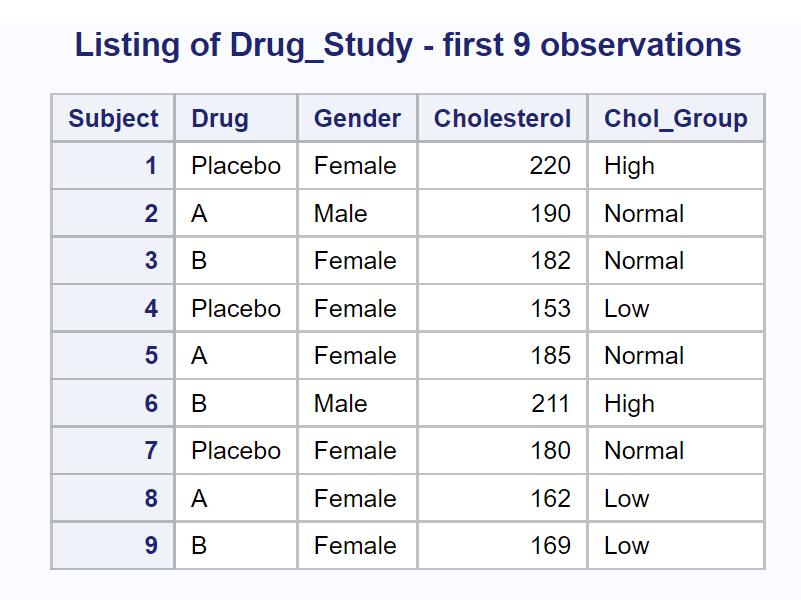Let's start out­ with the most basic summarization—computing statistics for all numeric variables for the entire data set. You can write a program as simple as:

```proc means data=Drug_Study; run;```

However, this program will compute default statistics for every numeric variable in the data set (including Subject). You will probably want to include PROC MEANS options to select what statistics you want and a VAR statement to list the variables that you want to summarize. Here is an example:

```title "Summarizing the Entire Drug_Study Data Set"; proc means data=Drug_Study n nmiss median mean std clm maxdec=3; var HR Cholesterol; run;```

The procedure options selected here are some of my favorites. This is what they represent:

 n The number of nonmissing observations nmiss The number of observations with missing values median The median value (the 50th percentile) mean The mean std The standard deviation clm The 95% confidence limits for the mean. (You are 95% confident that the mean from which this sample was taken is between these two limits.) maxdec The maximum number of places to print following the decimal point

Here is the output: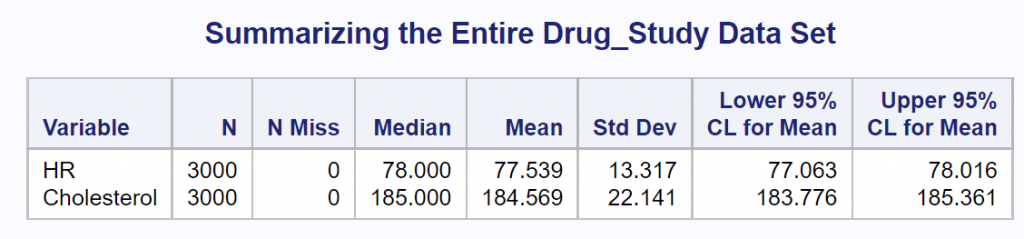You can tell this is simulated (fake) data because there are no missing values. This would be very unusual in a real-life situation.

Most researchers would like to break down the two variables of interest (HR and Cholesterol) by some of the classification variables, such as Gender and Drug. You use a CLASS statement to do this. Let's start out by requesting statistics on HR and Cholesterol, broken down by Drug.

```title "Variables Broken Down by Drug"; proc means data=Drug_Study n nmiss median mean std clm maxdec=3; class Drug; var HR Cholesterol; run;```

Here is the output: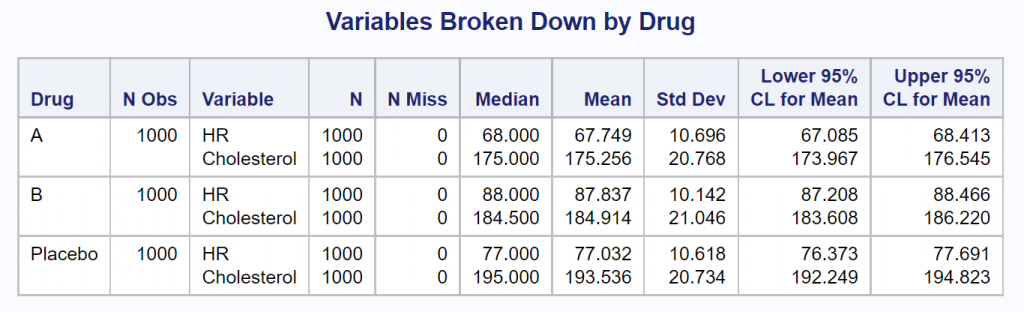You could repeat this program, substituting Gender instead of Drug as the CLASS variable, yielding the following: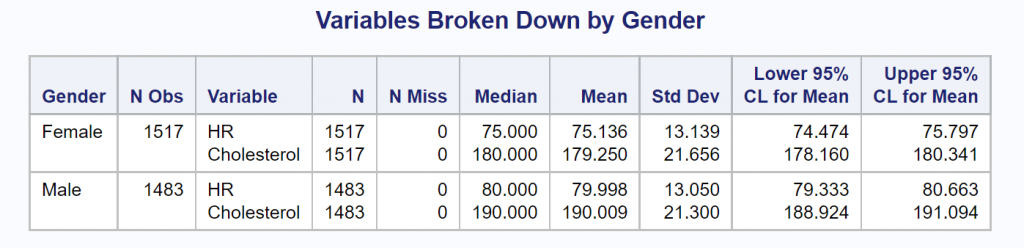Finally, you can include a CLASS statement, listing those two class variables. The program, modified to show statistics broken down by Drug and Gender is shown next, followed by the output.

```title "Variables Broken Down by Drug and Gender"; proc means data=Drug_Study n nmiss median mean std clm maxdec=3; class Drug Gender; var HR Cholesterol; run;```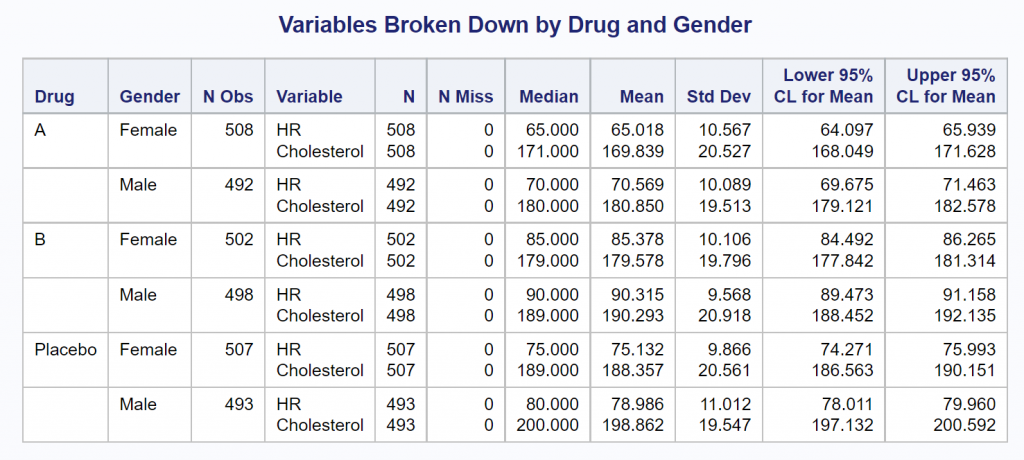It is tedious having to run PROC MEANS four times to produce all of the outputs shown thus far. Instead, you can use the two CLASS variables and add the PROC MEANS option PRINTALLTYPES when you run the program. It looks like this:

```title "Variables Broken Down by Drug and Gender"; footnote "Including the PROC MEANS option PRINTALLTYPES"; proc means data=Drug_Study n nmiss median mean std clm maxdec=3 printalltypes; class Drug Gender; var HR Cholesterol; run;```

In one run, you now see the two variables, HR and Cholesterol, for all combinations of the class variables as shown below.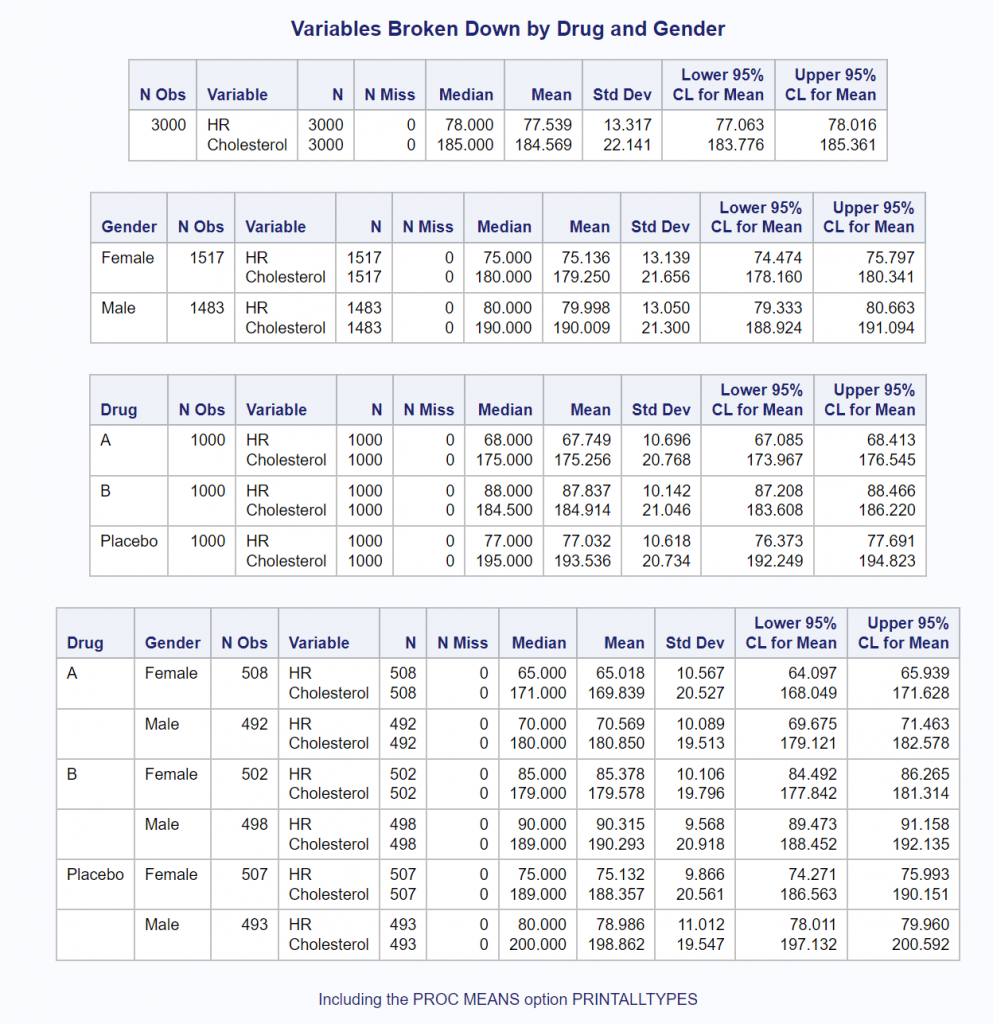All of the programs used in this blog were run using SAS OnDemand for Academics using the SAS Studio editor. You could have also produced all of the outputs using built-in SAS Studio tasks. You can read more about how to do this in my book A Gentle Introduction to Statistics Using SAS Studio in the Cloud.

As always, comments and/or corrections are welcome.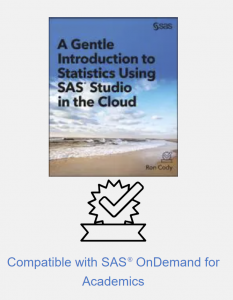Summarizing data was published on SAS Users.

This blog is a continuation of a previous blog that discussed creating simulated data sets. If you have not seen it, you might want to review it, especially if you are not familiar with the RAND function.

The program that I'm going to show you simulates a drug study with three groups (Placebo, Drug A, and Drug B), two genders, and correlated values of systolic blood pressure (SBP) and diastolic blood pressure (DBP).

```proc format; ❶ value \$Gender '0' = 'Male' '1' = 'Female'; run;   data Study; call streaminit(13579); ❷ do i = 1 to 30; do Drug = 'Placebo','Drug A','Drug B'; ❸ Subjec + 1; ❹ Gender = put(rand('Bernoulli',.5),1.); ❺ SBP = rand('Normal',160,10) - 20*(Drug = 'Drug A') ❻ - 30*(Drug = 'Drug B') - 5*(Gender = '1'); DBP = .5*SBP + 40*rand('Uniform'); ❼   SBP = round(SBP); ❽ DBP = round(DBP); output; end; end; drop i; format Gender \$Gender.; run;```

❶ Create a character format that will be used to format Gender.
❷ Because this is a drug study, you want to be able to reproduce the same random sequence at a later time. If you use the same argument in CALL STREAMINIT in another program, you will create identical random streams.
❸ Remember that SAS DO LOOPS can use character values. This is very handy.
❹ This SUM statement is generating the variable Subject.
❺ The Bernoulli distribution with the second argument set to .5, will assign gender (0 or 1) with a probability of .5 for both. The PUT function in this statement is doing a numeric-to-character conversion.
❻ This is a very interesting statement. You start by choosing values for SBP from a normal distribution with a mean of 160 and a standard deviation of 10. The logical expressions such as (Drug = 'Drug A') returns a 1 if the expression if true and 0 otherwise. By multiplying this by a numeric value, you can adjust the means in the three groups. The Placebo group will have a mean close to 160—the subjects in drug group A will be approximately 20 points lower than the Placebo group and the subjects in drug group B will be approximately 30 points lower than the Placebo group. Finally, females will be approximately 5 points lower than males.
❼ DBP will be correlated with SBP because it includes a proportion of SBP in the calculation. (See the previous simulated data blog for more details about generating correlated pairs.)
❽ Although I could have added the ROUND function when SBP and DBP were created, it makes the program a bit easier to read by rounding the values in separate statements.
The figure below shows the first nine observations in the simulated Study data set. This figure as well as all the box plots and the scatter plot that follow, were created using built-in SAS Studio tasks in the cloud version called SAS OnDemand for Academics.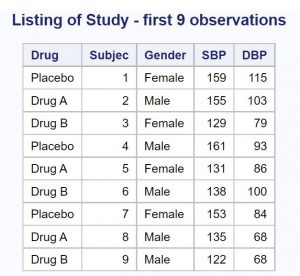The next figure is a box plot with SBP as the displayed variable. As a quick review, a box plot shows a vertical line at the median (in the middle of the box). The lower and upper edges of the box represent values at the 25th and 75th percentile respectively. The diamond in the box represents the mean. The distance between the 25th and 75th percentiles is called the inter-quartile range. Finally, any points more than 1.5 inter-quartile ranges below the 25th percentile or above the 75th percentile are plotted as circles and considered outliers. You can see one outlier in the placebo group in the left portion of the box plot below.

Notice that the means are approximately where you expect them to be (Placebo around 160, Drug A around 140, and Drug B around 130. The means are slightly lower because half of the subjects are female and their systolic blood pressures were designed to be approximately 5 points lower than the males.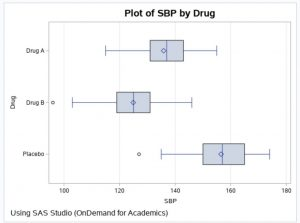Here is a plot of SBP by Gender.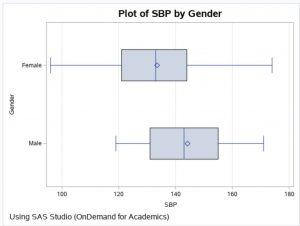The last figure is a plot of SBP by DBP. Notice that the values are correlated (the Pearson r is approximately .64).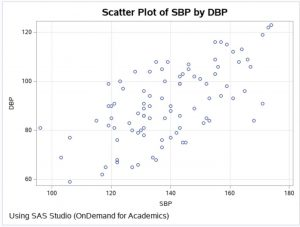Using techniques similar to this program, combined with methods in the previous simulation blog, you should be able to create your own custom simulated data sets.

Creating Simulated Data Sets (Part Two) was published on SAS Users.

There are times when it is useful to simulate data. One of the reasons I use simulated data sets is to demonstrate statistical techniques such as multiple or logistic regression. By using SAS random functions and some DATA step logic, you can create variables that follow certain distributions or are correlated with other variables. You might decide to simulate a drug study where the drug group has a higher or lower mean than a placebo group.

Because most programs that create simulated data use random numbers, let's start off by discussing the RAND function. This function can generate random numbers that follow distributions such as uniform, normal, Bernoulli, as well as dozens of other distributions. Veteran SAS programmers might be more familiar with some of the older random number functions such as RANUNI and RANNOR. RANUNI was used to generate uniform random numbers (numbers between 0 and 1) and RANNOR generated random numbers from a normal distribution. The RAND function has replaced all of the older functions and has a number of advantages over the older functions.

The first argument of the RAND function is the name of the distribution that you want to use, such as Uniform, Normal, or Bernoulli. For some of the distributions, such as Normal, you can supply parameters such as the mean and standard deviation. Here are some examples:

 Function Description rand('Uniform') Generates uniform random numbers (between 0 and 1) rand('Normal',100,20) Generates values from a normal distribution with a mean of 100 and a standard deviation of 20 rand('Bernoulli',.4) Generates a 0 or 1 with a probability of a 1 equal to .4 rand('Binomial',.2,5) Generates random numbers that represent the number of successes in a sample of size 5 with the probability of success equal to .2

Important Note: if you want a reproducible series of random numbers using the RAND function, you must seed it by a call to STREAMINIT (with a positive integer argument) prior to its use. For example:

` call streaminit(132435);`

To clarify the note above, here are two programs that use the RAND function—one with, and one without the call to Streaminit.

``` data Without; do i = 1 to 5; x = rand('Uniform'); output; end; drop i; run;```

Here is the output from running this program twice.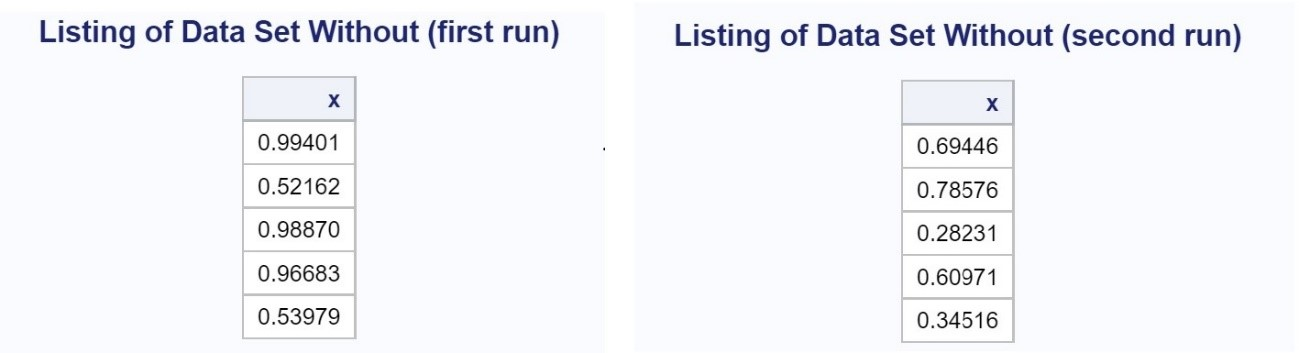Notice that the values of x are different in each run. Now let's run the same program with CALL STREAMINIT included. Here is the program.

```data With; call streaminit(13579); do i = 1 to 5; x = rand('Uniform'); output; end; drop i; run;```

And here are the output listings from running this program twice.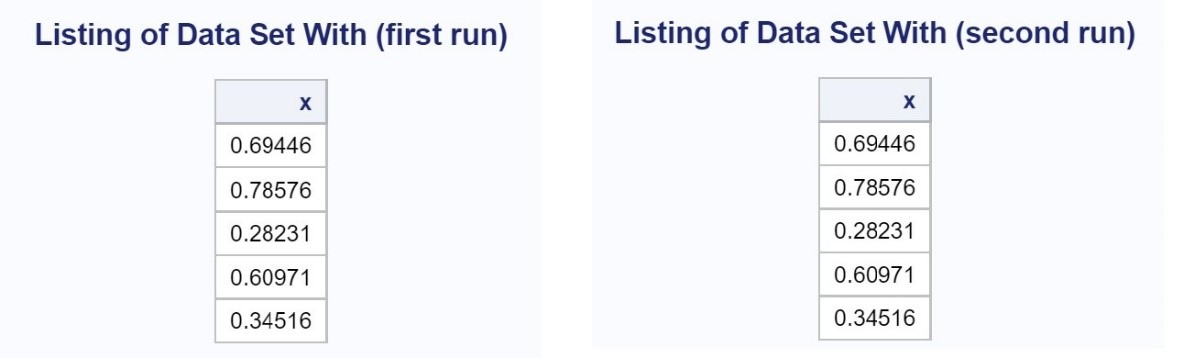Adding CALL STREAMINIT creates the same sequence of random numbers each time the program is run. This is useful if you are generating groups for a drug study and want to be able to re-create the random sequences when it comes time to break the blind and analyze the results. Another reason I sometimes want to generate a repeatable sequence of random numbers is for problem sets included in many of my books—I want the reader to get exactly the same results as I did.

Let's switch topics and see how to write a program where you want to simulate flipping a coin. The program below uses a popular method, but not it is not as elegant as the next program I'm going to show you.

```*Old fashioned way to generate "random" events; data Toss; do n = 1 to 10; if rand('uniform') lt .5 then Result = 'Tails'; else Result = 'Heads'; output; end; run;```

In the long run, half of the uniform random numbers will be less than .5, and the proportion of heads and tails will be approximately .5. Here is a listing of data set Toss.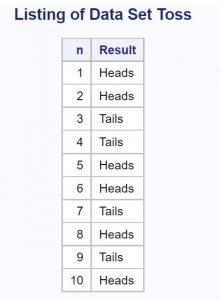A more sophisticated approach takes advantage of the RAND function's ability to generate random number from multiple distributions. A Bernoulli distribution is similar to a coin toss where you can adjust the probability of a 1 or 0 by including a second parameter to the function. The Toss2 program, shown below, does just that.

```*More sophisticated program; proc format; value Heads_Tails 0="Heads" 1="Tails"; run;   data Toss2; do n = 1 to 10; Results = rand('Bernoulli',.5); format Results Heads_Tails.; output; end; run;```

The format Heads_Tails substitutes the labels "Heads" and "Tails" for values of 0 and 1, respectively. Here is a listing of data set Toss2.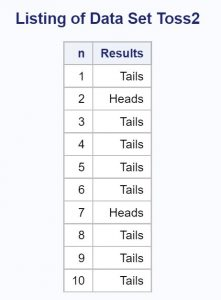The final discussion of this blog, concerns generating random values of two or more variables that are correlated. The example that follows generates x-y pairs that are correlated.

```*Creating correlated x-y pairs; data Corr; do i = 1 to 1000; x = rand('normal',100,10); y = .5*x + rand('Normal',50,10); output; end; drop i; run;```

By including a proportion of the x-value when creating the y-value, the x- and y-values will be correlated. Shown below is the output from PROC CORR, showing that x and y are correlated (r = .45586).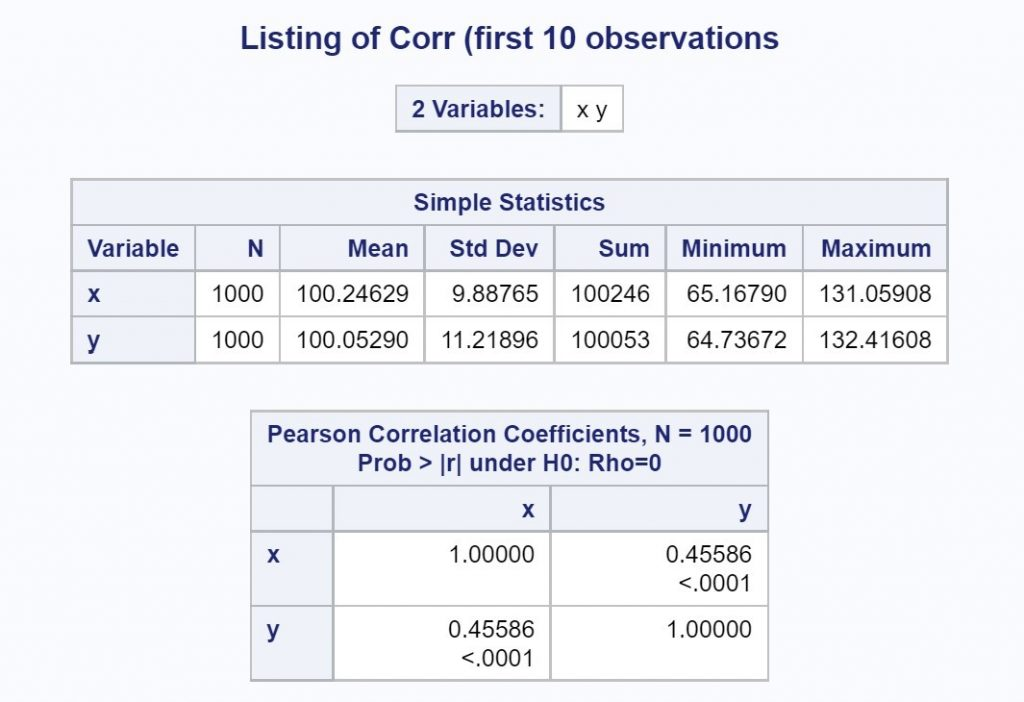I used a SAS Studio task to create the scatterplot shown next.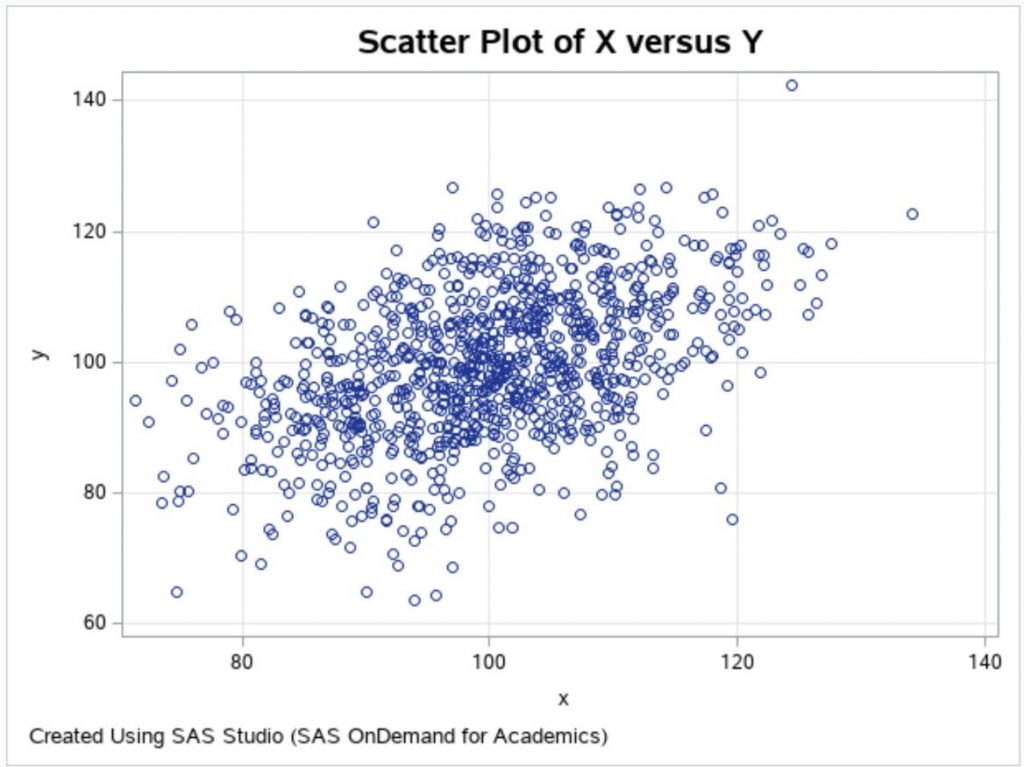You can increase or decrease the correlation by increasing the proportion of x used to create y. For example, you could use y = .8*x + rand('Normal',20,10); to create x-y pairs with a higher correlation.

You can see more examples of the RAND function in my book, SAS Functions by Example, Second Edition, available as an e-book from RedShelf or in print form from Amazon.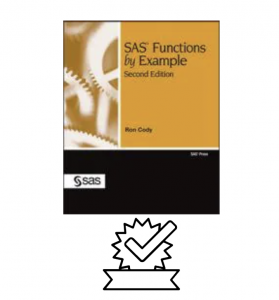To learn more about how to use SAS Studio as part of OnDemand for Academics, to write SAS programs, or to use SAS Studio tasks, please take a look at my new book: Getting Started with SAS Programing: Using SAS Studio in the Cloud (available in e-book from RedShelf or in a paper version from Amazon).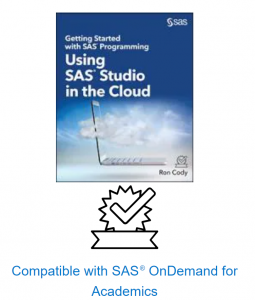I hope you enjoyed reading this blog and, as usual, I invite comments and/or questions.

Creating Simulated Data Sets was published on SAS Users.There are many reasons why you might want to encrypt data. I use a SAS program to encrypt a list of logon names and passwords. Before we get started describing how to encrypt data, let's discuss some basic concepts concerning encrypting and decrypting data.

All computer data is stored as a series of 1s and 0s. For example, an uppercase A in ASCII is 01000001. Many encrypting schemes use a key to transform plaintext into ciphertext. As an example, suppose your key is B (01000010).

I'm sure you remember the Boolean operators AND, NOT, and OR. If you perform an AND operator on A and B, the result is a 1 if both values are true (1) and false (0) otherwise. So, A AND B is 01000000. The OR operator results in a value of true if either A or B is true, or both A and B are true. Therefore, A OR B is 01000011. The operator that you might not be as familiar with is the exclusive OR (XOR) operator. This is similar to an OR operator except that if both A and B are true, the result is false. A XOR B is equal to 00000011. Why is this useful? An interesting property of the XOR operator is if you take the result and use the XOR operator again on the previous result, you get back to the original value.

The table below shows how the XOR operator works:

 A 0 1 0 0 0 0 0 1 B 0 1 0 0 0 0 1 0 A XOR B 0 0 0 0 0 0 1 1 (A XOR B) XOR B 0 1 0 0 0 0 0 1

If A is the cleartext message and B is the key, A XOR B is the ciphertext. If you perform an exclusive OR with the key (B) and the ciphertext (as was done in the last line), you get back the cleartext value (A).

Of course, you don't use single letter keys to encode messages. Key lengths of 8, 16, or even up to 512 are common. The problem with any key is that if you apply it to a long message, there is a pattern in the ciphertext that allows a code breaker to figure out how long the key is, and even what it is. There are several computer programs that can decode many of the popular encrypting methods. My favorite is:

https://www.geocachingtoolbox.com/

Here is a list of ciphers that can be broken with this program:

 ADFGX/ADFGVX cipher Four-square cipher Substitution cipher Affine cipher Gronsfeld cipher Trifid cipher Atbash cipher Kamasutra cipher Vanity code Bacon cipher Kenny code Vigenère cipher Bifid cipher One-time pad Vigenère cipher decoder Burrows-Wheeler transform Playfair cipher Caeser cipher (ROT13) Rail Fence cipher

Let's write a short SAS program that uses a key to encode a text string.

```data Encode;   retain Key 12345; ❶   length Letter \$ 1; ❷   String = 'This is a test';   do i = 1 to lengthn(String);   Letter = substr(String,i,1); ❸   Rank = rank(Letter); ❹   Coded = bxor(Rank,Key); ❺   Decoded = bxor(Coded,Key); ❻   Clear = byte(Decoded); ❼   output;   end;   drop i;   run;       title "Listing of Data Set Encode";   proc print dta=Encode noobs;   var Letter Key Rank Coded Decoded Clear;   format Key Rank Coded binary8.;   run;```

❶ A RETAIN statement is used to assign the number 12345 to a numeric variable called Key.  (You could have used an assignment statement, but using a RETAIN statement is more efficient and elegant.

❷ The variable Letter will hold each letter of the message and is set to a length of one.

❸ The SUBSTR function will extract each letter from String.

❹ Because Boolean operators only operate on true/false values, you use the RANK function to convert each letter to its ASCII value (stored internally as a series of 0s and 1s).

❺ You now use the BXOR (binary exclusive OR) function to encode each letter of your message.

❻ To demonstrate that the program is working, you use the BXOR function again to demonstrate that this process will return the original String.

❼ The BYTE function takes an ASCII value and returns the appropriate character.

Here is the listing of data set Encode: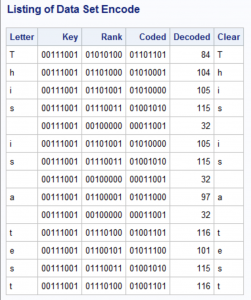Because this encryption method uses a single (and short) key, it would be fairly easy to break. What if you encode every letter of the original message with a different key? You can accomplish this by using the SAS random function RAND and using a seed value so that the same series of random numbers gets generated every time you run the program. You can even use one of a dozen different random distributions, to make it harder for someone to decode your file. Here is an example:

First, here is a copy of my text file that contains my secret message (stored in the location c:\Books\Blogs\Cipher\Clear_Text.txt).

Good morning Mr. Phelps.

Your mission, should you decide to accept it,

is to rid the world of evil.

As usual, if you or any member of your team are caught or killed,

the Secretary will disavow any knowledge of your actions.

The following program encrypts this file and creates a temporary data set (in a real situation, you would make this a permanent data set):

```data Coded;   call streaminit(13579); ❶   array l \$ 1 _temporary_; ❷   array num _temporary_; ❸   array xor; ❹   infile "c:\Books\Blogs\Cipher\Clear_Text.txt" pad;   input string \$150.; ❺   do i = 1 to dim(l);   l[i] = substr(string,i,1); ❼   num[i] = rank(l[i]); ❽   xor[i] = bxor(num[i],int(100*rand('Uniform'))); ❾   end;   keep xor1-xor150;   run;```

❶ You need to set a seed value using CALL STREAMINIT so that when you run the decoding program, you will generate the same series of random numbers.

❷ This temporary array will hold up to 150 characters.

❸ The Num array holds the numerical ASCII value for each of the characters in the line.

❹ The XOR array holds the values of the exclusive OR between each numerical ASCII value and the Key.

❺ Read in a string of up to 150 characters.

❻ The DO LOOP picks up each character in a line, starting from 1 and ending at the length of each line.

❼ The RANK function outputs the ASCII value of each character.

❽ The BXOR (binary exclusive OR) function performs the exclusive OR between each ASCII value and the Key.

To decode this message, use the following program:

```data Decode;   call streaminit(13579); ❶   array l \$ 1 _temporary_;   array num _temporary_;   array xor;   length String \$ 150;   set Coded;   do i = 1 to dim(l);   num[i] = bxor(xor[i],int(100*rand('Uniform'))); ❷   l[i] = byte(num[i]); ❸   substr(String,i,1) = l[i]; ❹   end;   keep String;   run;```

❶ Notice that the value of the CALL STREAMINIT routine uses the same seed as the previous program.

❷ The BXOR function between each coded value and the Key, will produce the cleartext.

❸ The BYTE function will convert the ASCII values back to letters, numbers, and other characters.

❹ Finally, the SUBSTR function used on the left-hand side of the equal sign will place each of the characters into the appropriate location in the String variable. (See my previous blog that discusses the use of the SUBSTR function used on the left-hand side of the equal sign.)

Here is the output: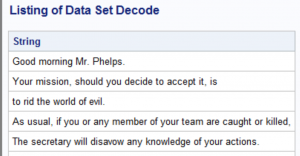It would be straightforward to convert these two programs into macros so that you could encrypt and decrypt any file.

Of course, I would be remiss if I didn't mention that you can encrypt a SAS data set using two data set options ENCRYPT=and ENCRYPTKEY="password". But what would be the fun of that?

Here is an example:

```*Note: AES stands for Advanced Encryption Standard   If you use quotation marks on the ENCRYPTKEY= option, you have   more flexibility in choosing a password (maximum length=64);       data Secret(encrypt=aes encryptkey="mypassword");   input String \$80.;   datalines;   This is a secret message.   See if you can decode it.   This message will not self-destruct!   ;```

You can decode the encrypted data set by including the DATA set option ENCRYPTKEY="password" in any procedure, such as the PROC PRINT shown below:

```title "Listing of Data Set Secret";   proc print data=Secret(encryptkey="mypassword") noobs;   run;```

Encrypting Data Using SAS was published on SAS Users.